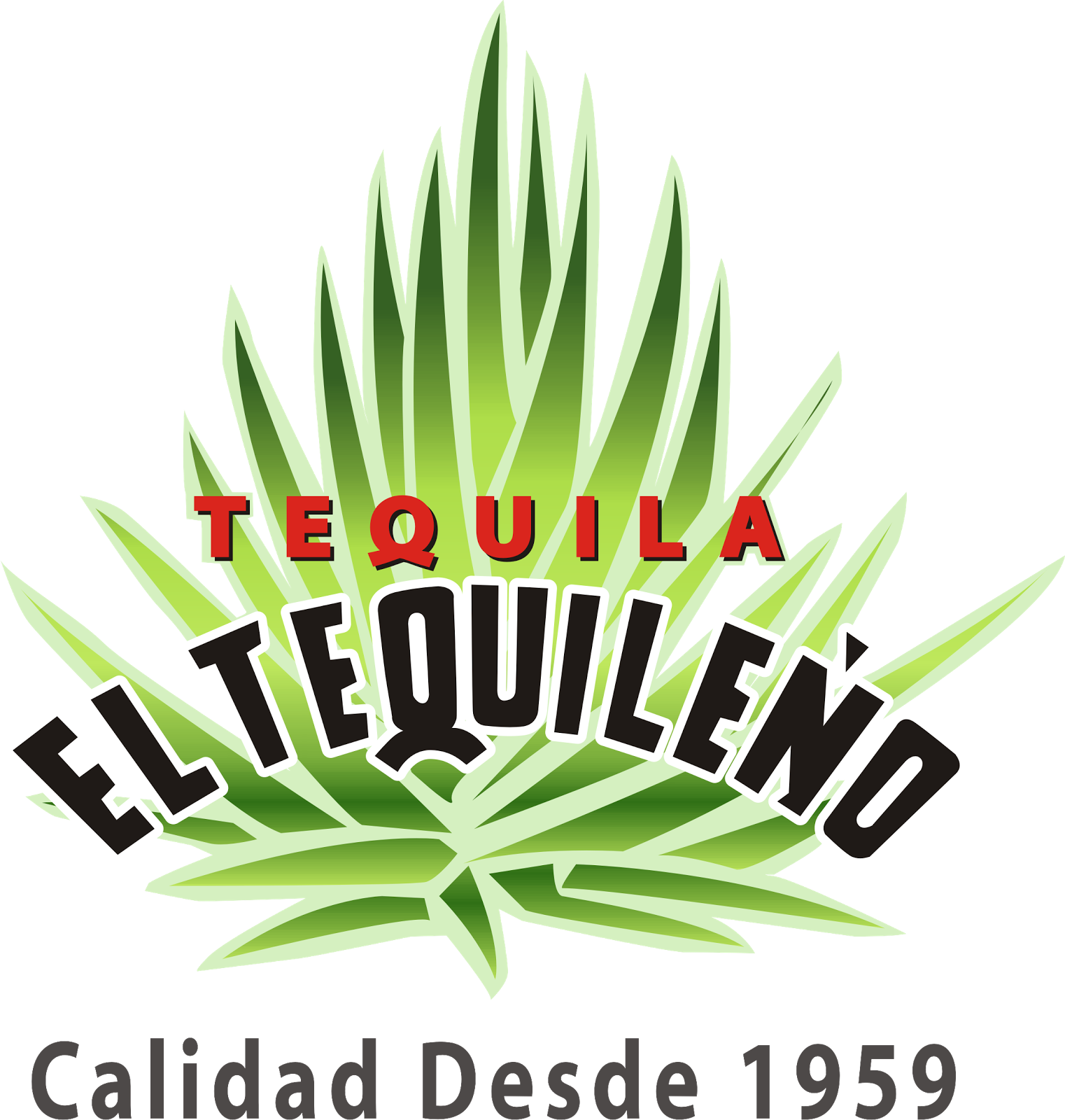# Mathematical model

In order to further understand our design we are proposing two mathematical models which determine different variables that will help in the optimization and efficiency necessary for the production of PHA.

## Mathematical model 1

The first mathematical model is an adequation of the one proposed by Patwardhan and Srivastava about the cultivation of R. eutropha and it's biopolymer production in order to model that of Pseudomona putida and its production of PHA. This model will not only facilitate the understanding of the system but also help in designing the nutrient-limited fed-batch cultivation-(s) to improve the productivity.

Basic assumptions of the model were:

1.- Biomass (X) is structured as having two components:

a) The catalytically active component consisting of proteins and nucleic acids (R).

b) The inert component which is the product PHA (P)

2.- Nitrogen is the limiting substrate affecting the growth kinetics.

### Nomenclature

Ks1= saturation constant for monod growth term.

Ks2= saturation constant for sigmoidal growth term.

K1= constant for non-growth associated term.

K2= constant for inhibition due to high product concentration.

e= kinetic parameter for growth associated term.

f= kinetic parameter.

a, b, c, = kinetic parameters.

m= exponent in inhibition term.

n= exponential constant for sigmoidal growth.

P= product concentration

R= residual biomass concentration.

Rmin= minimum value of R at which PHA accumulation starts

SG= glucose concentration

Sm= ratio of N/C at which specific growth rate is zero

SN= nitrogen concentration

X = R + P

Rate of formation of R is given as: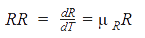The specific growth rate equation featured nutrient limitation by both Monod and sigmoidal growth terms. The inhibition due to N/C ratio (studied by Mulchandani et. al) was also incorporated in the model structure: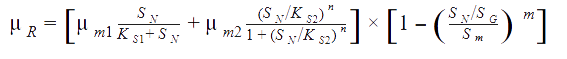The rate of formation of product (P) was assumed to have growth and non-growth associated components, and inhibition due to high product concentration: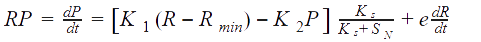Glucose consumption rate was assumed to be due to growth of R, formation of P and maintenance (proportional to R):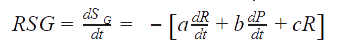Nitrogen was consumed due to the growth of the catalytically active biomass (R):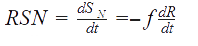### Experimental behavior obtained during PHA production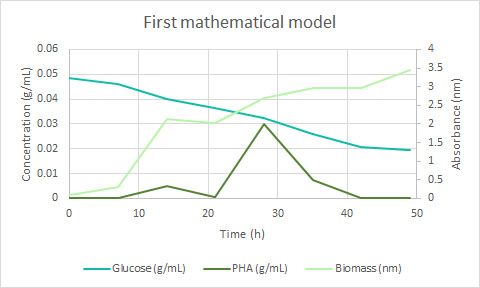## Mathematical model 2

Acetyl-CoA carboxylase (ACC) is a complex multifunctional enzyme system which catalyzes the carboxylation of acetyl-CoA to malonyl-CoA, the rate-limiting step in fatty acid synthesis. There are two ACC forms, alpha and beta, encoded by two different genes. ACC-alpha is highly enriched in lipogenic tissues.

The enzyme is under long term control at the transcriptional and translational levels and under short term regulation by the phosphorylation/dephosphorylation of targeted serine residues and by allosteric transformation by citrate or palmitoyl-CoA.

The efficiency of overexpressed Acetyl-CoA carboxylase will be mathematically estimated to convert Acetyl-CoA to Malonyl-CoA during the PHA production step to prevent the bacteria from targeting the Krebs cycle and improve PHA performance.

For an allosteric enzyme, as the ACC, Cornish-Bowden described the MWC model as follows:

### Formulas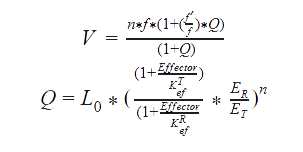### Nomenclature

f = the rate equation for a single subunit in Relax-state.

f' = the rate equation for a single subunit in Tense-state.

Q = function that determines the ratio between R and T forms of the enzyme.

ER = the concentration of an enzyme without substrates and products in R-state.

ET = the concentration of an enzyme without substrates and products in T-state.

L0 = the constant of transition between R and T states of the enzyme.

Effector = the concentration of the allosteric effector.

KefR = the constant of dissociation of the effector for R form of the enzyme.

KrfT= the constant of dissociation of the effector for T form of the enzyme.

n = the number of subunits of the enzyme.

Some adaptations were made from the Peskov method for our enzyme, and the solution for the equation, in the R-state (for T-state the equations are solve in the same way, but with the corresponding constants), goes as follows:

### Formulas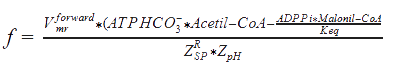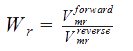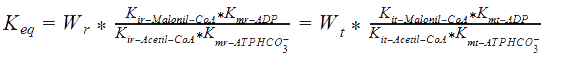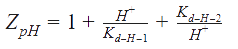### Nomenclature

Kmr_ATPHCO, Kmr_Acetil-CoA, Kmr_ADP, and Kmr_Malonil-CoA = Michaelis constants of substrates and products.

Kir_Acetil-CoA, Kir_ADP, and Kir_Malonil-CoA, Kir_ATP4+ = Constants of dissociation of substrates.

Kd_H_1 and Kd_H_2 = Constants of proton binding in the active center of the enzyme.

Vmrforward and Vmrreverse = Maximum rates of forward and reverse reactions.

WR = The ratio of maximum rates of forward and reverse reactions at R-state of the monomer.

Keq = The equilibrium constant of the reaction.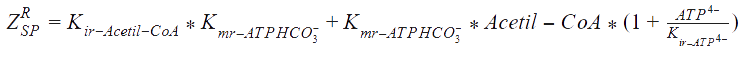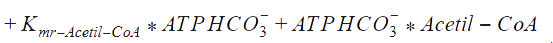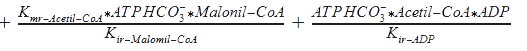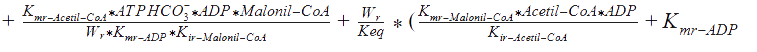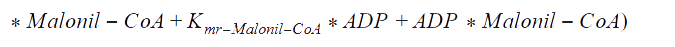The following functions have been derived according to the main regulatory features of the Acetyl Co-A carboxylase, taking into account the allosteric effectors. According to the reference, assumptions were made, as that citrate and glutamate bind to the same regulatory side, while AMP binds to another:

### Formulas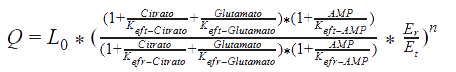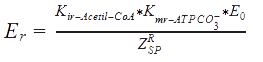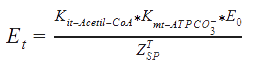### Nomenclature

E0 = The total concentration of the enzyme.

Now, the rate equation of the MWC model can be solved substituting the past equations on it.

##### References

NCBI. (2017). ACACA acetyl-CoA carboxylase alpha [Homo sapiens (human)]. Recovered september 29 at https://www.ncbi.nlm.nih.gov/gene/31

Patwardhan, P. R. and Srivastava, A. K. (2004). Model-based fed-batch cultivation of R. eutropha for enchanced byopolumer production. Recovered from http://www.eprint.iitd.ac.in/bitstream/2074/518/1/patwardhanmod2004.pdf

Peskov, K., Goryanin, I. and Demin, O. (2008). Kinetic model of phosphofructokinase-1 from Escherichia coli. Journal of Bioinformatics and Computational Biology. Recovered from: http://www.worldscientific.com/doi/abs/10.1142/S0219720008003643